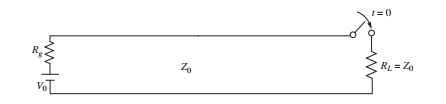### Create an Account

Home / Questions / In Figure 1039 RL Z0 and Rg Z03 The switch is closed at t 0 Determine and plot as funct...

# In Figure 1039 RL Z0 and Rg Z03 The switch is closed at t 0 Determine and plot as functions of time a the voltage across

In Figure 10.39, RL = Z0 and Rg = Z0/3. The switch is closed at t = 0. Determine and plot as functions of time (a) the voltage across RL ; (b) the voltage across Rg; (c) the current through the batteryJun 13 2020 View more View LessSubscribe To Get Solution